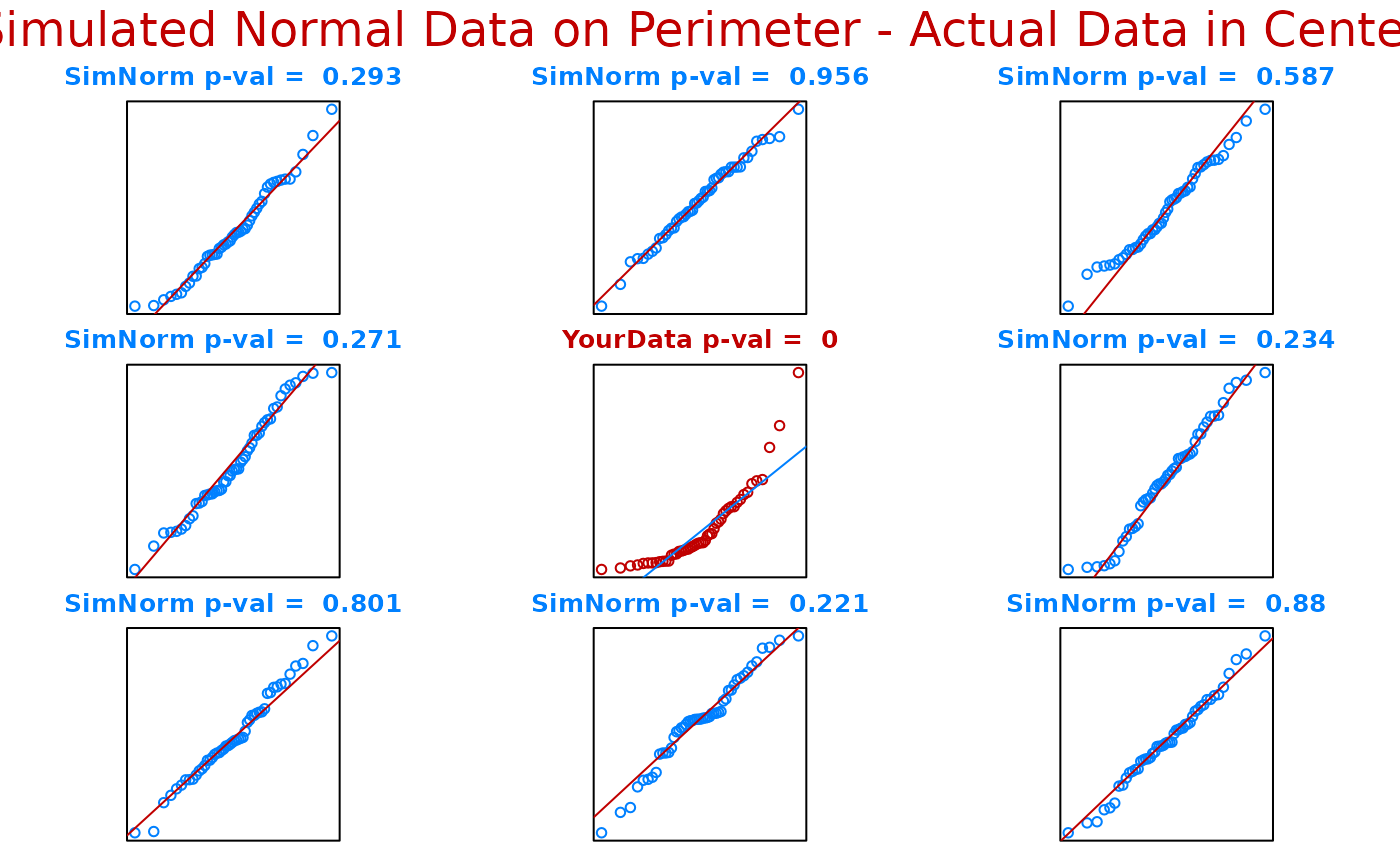Q-Q plots of randomly generated normal data of the same size as the tested data are generated and ploted on the perimeter of the graph while a Q-Q plot of the actual data is depicted in the center of the graph.

ntester(actual.data)

## Arguments

actual.data

a numeric vector. Missing and infinite values are allowed, but are ignored in the calculation. The length of actual.data must be less than 5000 after dropping nonfinite values.

## Details

Q-Q plots of randomly generated normal data of the same size as the tested data are generated and ploted on the perimeter of the graph sheet while a Q-Q plot of the actual data is depicted in the center of the graph. The p-values are calculated form the Shapiro-Wilk W-statistic. Function will only work on numeric vectors containing less than or equal to 5000 observations.

Alan T. Arnholt

## Examples


ntester(rexp(50,1))# Q-Q plot of random exponential data in center plot
# surrounded by 8 Q-Q plots of randomly generated
# standard normal data of size 50.